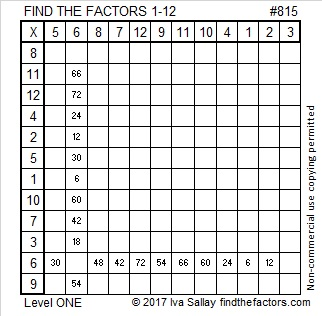# 815 and Level 1

Since multi-digit 815 ends with 5, it is a composite number, and it is also the hypotenuse of a Pythagorean triple:

• 489-652-815 which is 163 times 3-4-5.

Can you write the numbers 1 – 12 in both the first column and the top row so that this puzzle functions like a multiplication table?

Print the puzzles or type the solution on this excel file: 12 factors 815-820

• 815 is a composite number.
• Prime factorization: 815 = 5 x 163
• The exponents in the prime factorization are 1 and 1. Adding one to each and multiplying we get (1 + 1)(1 + 1) = 2 x 2 = 4. Therefore 815 has exactly 4 factors.
• Factors of 815: 1, 5, 163, 815
• Factor pairs: 815 = 1 x 815 or 5 x 163
• 815 has no square factors that allow its square root to be simplified. √815 ≈ 5482048.